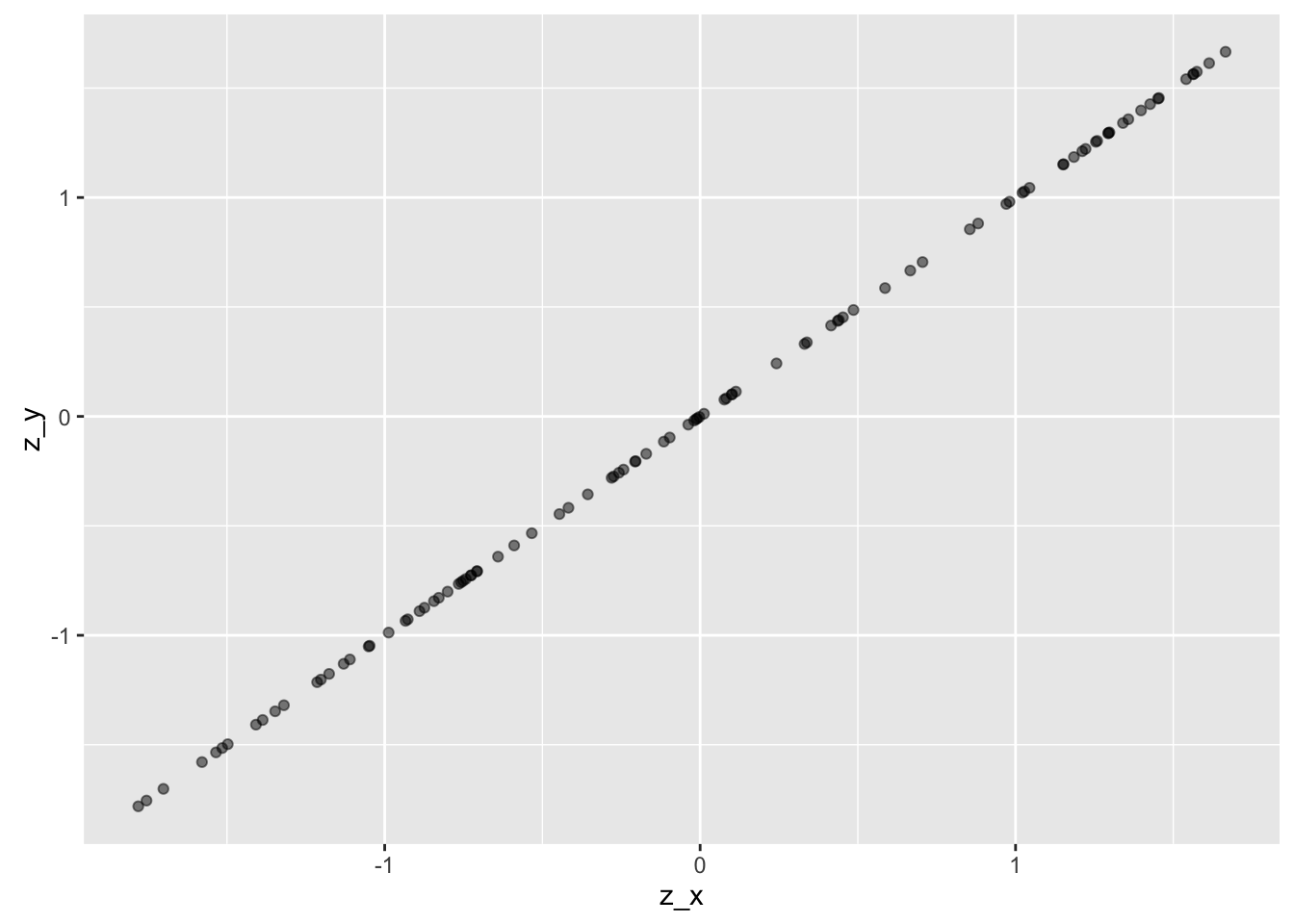# Why absolute correlation value (r) cannot exceed 1. An intuition.

## August 28, 2016

Reading time ~6 minutes

Pearson’s correlation is a well-known and widely used instrument to gauge the degree of linear association of two variables (see this post for an intuition on correlation).

There a many formulas for correlation, but a short and easy one is this one:

$r = \varnothing(z_x z_y)$.

In words, $r$ can be seen as the average product of z-scores.

In “raw values”, r is given by

$r = \frac{\frac{1}{n}\sum{\Delta X \Delta Y}}{\sqrt{\frac{1}{n}\sum{\Delta X^2}} \sqrt{\frac{1}{n}\sum{\Delta Y^2}}}$.

Here, $\Delta$ refers to the difference of a value to its mean.

At times it is helpful to write r so that the similarity to the covariance gets clear:

$r = \frac{Cov(X,Y)}{sd(X) \cdot sd(Y)}$

OK. But why is it, that r cannot be larger than 1 (and not smaller than -1)?

That is, why does the following inequality hold: $-1 \le r \le 1$?

This is the question we are addressing in this post. I haven’t found much on that on the net, so that’s why I sum up some thoughts in this post.

## Cauchy-Schwarz Inequality

One explanation, quite straight forward, is based on the Cauchy-Schwarz Inequality. This inequality can be stated as follows:

${\langle x,y \rangle}^2 \le \langle x,x \rangle \cdot \langle y,y \rangle$,

where $\langle x \rangle$ denotes the dot product of a vector, eg. x.

This formula can be rewritten as

$(\sum{x_i y_i})^2 \le (\sum(x^2_i)) \cdot (\sum(y^2_i))$

But this formula looks very similar to the correlation, if we first take the square root of both sides:

$(\sum{x_i y_i}) \le \sqrt{(\sum(x^2_i))} \cdot \sqrt{(\sum(y^2_i))}$

and then cancel out the (1/n) part from the raw values equation of r above:

$r = \frac{\sum{\Delta X \Delta Y}}{\sqrt{\sum{\Delta X^2}} \sqrt{\sum{\Delta X^2}}}$.

Now rewrite r in this way:

$\sum{\Delta X \Delta Y} \le \sqrt{\sum{\Delta X^2}} \sqrt{\sum{\Delta Y^2}}$.

The raw formula of r matches now the Cauchy-Schwarz inequality! Thus, the nominator of r raw formula can never be greater than the denominator. In other words, the whole ratio can never exceed an absolute value of 1.

## Looking at the regression line

A second line of reasoning why r cannot the greater than 1 (less than -1) is the following.

Suppose X and Y are perfectly correlated, for example like this:

x <- runif(n = 100, min = 0, max = 10)
res <- rnorm(n = 100)
y <- x
library(ggplot2)
library(tibble)
ggplot(tibble(x, y), aes(x = x, y = y)) + geom_point(alpha = .5)Now, what happens if we z-scale X and Y? Not much:

z_x <- as.numeric(scale(x))
z_y <- as.numeric(scale(y))

df1 <- tibble(z_x, z_y)
ggplot(df1, aes(x = z_x, y = z_y)) + geom_point(alpha = .5)The difference is that mean X and meany Y is both zero, and SD for both X and Y is 1, so the scaling has changed (the line has a gradient of 1 now).

Note that perfect correlation of z-scaled variables means that for each $X_i$ there is an $Y_i$ of same value.

For example:

library(magrittr)
z_x <- x %>%  scale %>% as.numeric %>% sort
z_y <- y %>%  scale %>% as.numeric %>% sort
df2 <- tibble(z_x, z_y)
head(df2)

## # A tibble: 6 x 2
##         z_x       z_y
##       <dbl>     <dbl>
## 1 -1.688960 -1.688960
## 2 -1.688875 -1.688875
## 3 -1.685675 -1.685675
## 4 -1.621873 -1.621873
## 5 -1.554167 -1.554167
## 6 -1.532031 -1.532031


But if $X_i = Y_i$ for all X and Y it means that the means and variances of X and Y are the same, too. It actually means that X equals Y.

If we look at the formula of the correlation for perfectly correlated z-scaled variables X and Y we find:

$r_z = \frac{Cov(X,Y)}{sd(X) \cdot sd(Y)} = \frac{Cov(X,X)}{sd(X) \cdot sd(X)} = \frac{Var(X)}{Var(X)} = 1 = \frac{Var(Y)}{Var(Y)}$

In words, when two variables are perfectly correlated (ie., their graph is a line), then r=1.

### This blog has moved

This blog has moved to Adios, Jekyll. Hello, Blogdown!… Continue reading

#### Wie gut schätzt eine Stichprobe die Grundgesamtheit?

Published on November 17, 2017

#### Some thoughts on tidyveal and environments in R

Published on November 16, 2017